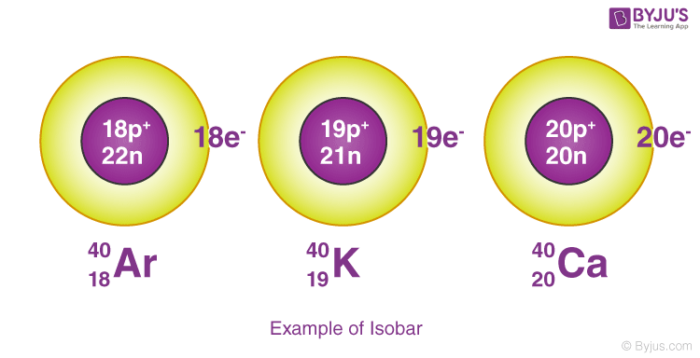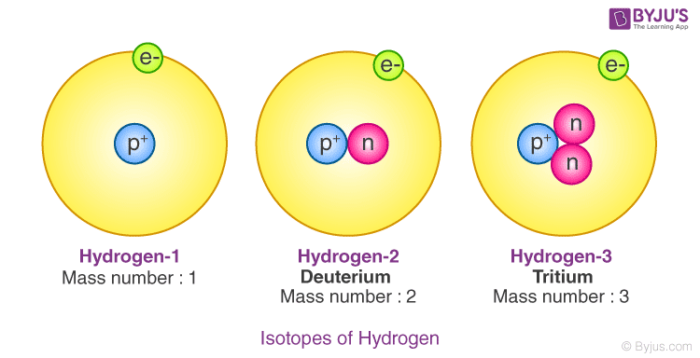# Isotopes and Isobars

Isobar are elements that differ in chemical properties but have the same physical property. So, we can say that isobars are those elements that have a different atomic number but the same mass number. In contrast, Isotopes are those elements having the same atomic number and different mass numbers.

## What are Isobars?

Isobars are atoms (nuclides) of different chemical elements which differs in the chemical property but has the same physical property. So, we can say that isobars are those elements which have a different atomic number but the same mass number. Their chemical property is different because there is a difference in the number of electrons. It has the same atomic mass but different atomic no. This is because an additional number of neutrons compensates for the difference in the number of nucleons.

The example of two Isotopes and Isobars is iron and nickel. Both have the same mass number which is 58 whereas the atomic number of iron is 26, and the atomic number of nickel is 28.## What are Isotopes?

Let us take an example of two things which have the same colour, same physical appearance, such that you cannot distinguish between these two. But when you measure the weight of these two things then you find that they are different. You can relate the concepts of isotopes with this example.

As we all know that atoms are made up of electrons, protons, and neutrons. The nucleus is made up of protons and neutrons and the electrons revolve around the nucleus. Atomic mass is the sum of some protons and the number of neutrons and atomic number is equal to the number of protons. In an element, the number of protons is always the same, but the number of neutrons keeps on changing.

Isotopes are the atoms in which the number of neutrons differs and the number of protons is the same. From the above definition of atomic mass and the atomic number, we can conclude that isotopes are those elements having the same atomic number and different mass numbers.

Let us know something about the isotopes of hydrogen: There are three isotopes of hydrogen and these are protium, deuterium, and tritium. All three of them have the same number of protons, but the numbers of neutrons differ. In protium the number of neutrons is zero, in deuterium, it is one and in tritium, the number of neutrons is two.## Difference between isotopes and isobars

The below table gives a few differences between isotopes and isobars.

 Isobars Isotopes Chemicals elements which have the same mass Different atomic structure of the same element The atomic masses are equal The atomic masses are different Often the physical properties are similar Usually, physical properties are different Atomic numbers are different Atomic numbers are the same Chemical elements are different Same chemical elements having different  mass numbers

For more content on isotopes and isobars register to BYJU’S – The Learning App.

## Frequently Asked Questions – FAQs

Q1

### Name three isotopes of oxygen.

16O, 17O, and 18O.

Q2

### How are isotopes formed?

Atoms that have an equal number of protons but a different number of neutrons and protons are called isotopes.

Q3

### Give some examples of stable isotopes.

Vanadium, carbon, calcium, potassium are stable isotopes.

Q4

### The unstable isotopes emit what rays?

Alpha, beta, and gamma rays are emitted by unstable isotopes.

Q5

### What are isobars?

Atoms which have the same mass number but a different number of protons are called isobars.

Q6

### Give some examples of isobars.

40S, 40Ar, 40Ca. 40Cl, and 40K.